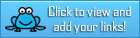# Tag Archives : #pbl

## April Teacher Talk

It’s April already, the school year is almost over.  Now it’s time to look forward toward spring vacation if it hasn’t already come and gone. Stop by our blog linky and see what great tips and advice are there just for you.

*******************************
Spring Activities
Begin with mud and splash into fun learning this Spring.
*******************************
Music to Calm the Soul and Enhance Learning
“Music is the electrical soil in
which the spirit lives, thinks and invents.” –Ludwig van Beethoven. Why
is it that for many of us, music is an essential part of our personal
lives yet when we go to work or school we turn it off?
*******************************
The Elephant in the Classroom
Where is the elephant in the classroom? The recent college admissions scandal might give us a clue.
*******************************
Scaredy Squirrel – Mentor Text
What happens when Scaredy Squirrel visits YOUR home? This free writing activity will get your writers excited!
*******************************
Do you know how to decide between the words bad and badly? Many people don’t.
*******************************
7 Tips to Help Students Revise Their Essay
Try out these 7 helpful tips to guide your students during the revising phase. Your writers can use these tips throughout during the writing process.
*******************************
How to Start Book Clubs in Your Classroom
Are you considering using book clubs in your classroom? Learn five things you should do before you introduce book clubs to your class.
*******************************
Make Mistakes on Purpose
When students have the opportunity to correct the teacher, it reinforces that language point, assesses the students’ understanding of that language point, gives the learner confidence, and teaches students to problem-solve.
*******************************
Exploring Patterns With Project-Based Learning: Rotations, Reflections & Translations

Explore 4th and 5th grade geometry concepts with project-based learning. It’s always surprising who excels with this type of project, and who finds them challenging. This one doesn’t disappoint!
*******************************
Whole Body Learning
Geometry was a daunting subject for me in high school. So when I started teaching, I was determined to think of a way to teach geometry that was fun and interactive.
*******************************
Be sure to visit all of our teachers for some awesome ideas to use with your students.

## February Teacher Talk

Posted by Deann Marin of Socrates Lantern

Welcome to our February Teacher Talk.  All of us from the Teacher Talk collaborative would like wish you a Happy Valentines Day.  We have so many fab things this month from  proof-reading ideas, to ELA to math activities, to celebrating Black History Month and President’s Day, you don’t want to miss reading these blog posts from some awesome educators. This month, we’re honored to have Daniel with us, as our  guest blogger.
If you’re interested in joining this unique group of teacher entrepreneurs and blogging buddies and our blog linky, sign up here…. If you decide to join, be sure to mention one of our names.
<<<<<<<<<<<<<<<<<<<<<<<<<<<<<<<<<<<<<<<<<<<<<<<<<<<<<<<<<<<
Navigating Life as a Single Dad
By Daniel of Dad Solo whose guest post can be found at Rainbow City Learning

Single parents have a difficult job, and even in the best of circumstances, it can be a struggle. Moms have it tough, but so do dads.
<<<<<<<<<<<<<<<<<<<<<<<<<<<<<<<<<<<<<<<<<<<<<<<<<<<<<<<<<<<
I Dreaded my Third Period Class
By Deann Marin of Socrates Lantern

It was cold and blustery outside, one of those days that you just wanted to stay under the covers. But it was only hump day and I had a busy schedule, so I dragged myself out of bed, had my cup of coffee and a piece of toast, jumped into my car and was on my way.
<<<<<<<<<<<<<<<<<<<<<<<<<<<<<<<<<<<<<<<<<<<<<<<<<<<<<<<<<<<

Is This Routine? Why Not?
By Susan Berkowitz of Susan Berkowitz

Research tells us that routines are the heart of language and symbbol development. Routines are a sequence of actions or events that are repeated over and over again.
<<<<<<<<<<<<<<<<<<<<<<<<<<<<<<<<<<<<<<<<<<<<<<<<<<<<<<<<<<<

By Sally Hansen of Purposeful Plans

Have you ever set a goal to cover everything you are required to teach during the school year?  Many teachers try to meet this goal each year and end up frustrated because we are short on time!  It is very difficult to get everything we are required to teach during the school year, take the cross-curricular approach!
<<<<<<<<<<<<<<<<<<<<<<<<<<<<<<<<<<<<<<<<<<<<<<<<<<<<<<<<<<<

Improving Class Cooperation
By Gini Musmanno of Reading Spotlight

Wishing and hoping, and thinking and praying, for a courteous and amenable class? Then give students a little nudge toward cooperation and affirmation of each other this month.
<<<<<<<<<<<<<<<<<<<<<<<<<<<<<<<<<<<<<<<<<<<<<<<<<<<<<<<<<<<

By Lisa Robles of LisaTeachR’s Classroom

<<<<<<<<<<<<<<<<<<<<<<<<<<<<<<<<<<<<<<<<<<<<<<<<<<<<<<<<<<<

Building Understanding, One Brick at a Time
By Kathie Yonemura of Tried & True Teaching Tools

Getting your hands muddy is a great way to learn! Social Studies is meaningful when students experience content learned.
<<<<<<<<<<<<<<<<<<<<<<<<<<<<<<<<<<<<<<<<<<<<<<<<<<<<<<<<<<<

How to Select Books for Your Classroom Book Clubs
By Marcy Howe of It’s a Teacher Thing

Read five essential tips for finding “just right” books for your classroom book clubs. The books you choose are crucial to running successful classroom book clubs.
<<<<<<<<<<<<<<<<<<<<<<<<<<<<<<<<<<<<<<<<<<<<<<<<<<<<<<<<<<<